## ML Aggarwal Class 8 Solutions for ICSE Maths Chapter 17 Visualising Solid Shapes Check Your Progress

Question 1.
Write the number of faces, vertices and edges of a
(i) hexagonal pyramid
(ii) octagonal pyramid
(iii) decagonal pyramid
(iv) nonagonal pyramid
(v) heptagonal prism
(vi) decagonal prism.
Solution: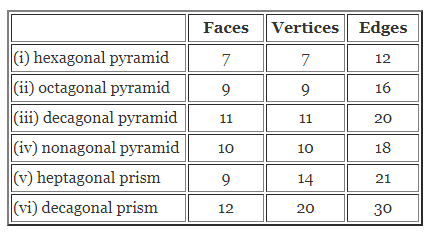Question 2.
Give three examples of 3-dimensional shapes around you which are the combinations of 2 or more 3-dimensional shapes.
Solution: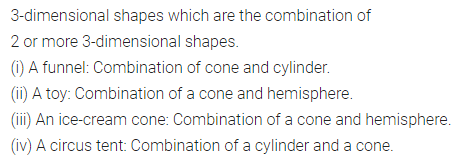Question 3.
Give two examples of solids which are not polyhedrons.
Solution:Question 4.
Why a pentagonal pyramid having all its edges congruent cannot be a regular polyhedron?
Solution:Question 5.
In a polyhedron, if F = 8 and V = 12 then find the number of edges.
Solution: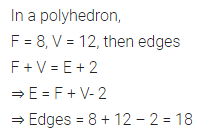Question 6.
Verify Euler’s formula for the following figures: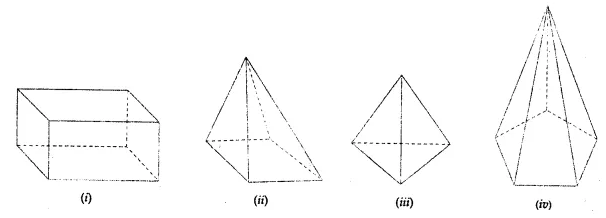Solution:Question 7.
For each of the given solid, the three views are given. Identify for each solid the corresponding top, front and side views: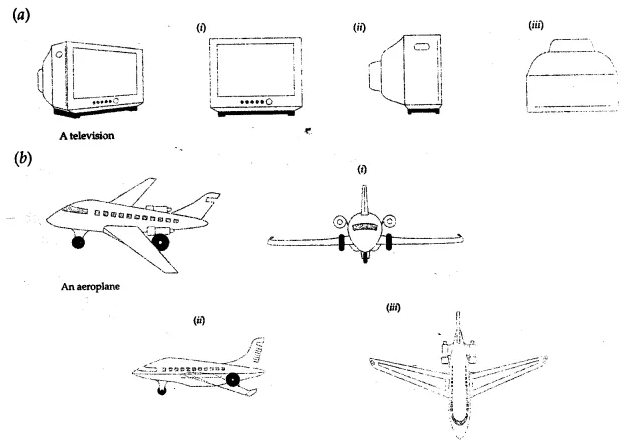Solution: